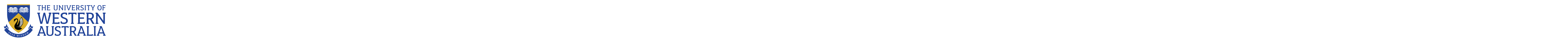CITS2002 Systems Programming

# Department of Computer Science and Software Engineering

## CITS2002 Systems Programming

### Labsheet 2 - for the week commencing 12th August 2019

(Clarifying something from Labsheet-1) many exercises will require you to write functions that take one or more arguments and perform operation on them. Typically, these arguments will be read from the command-line of the program. The name of your program may be obtained from the variable (array element) argvalue. The first "true" command-line argument to your program may be obtained from the variable (array element) argvalue. Subsequent command-line arguments may be obtained with the code argvalue, argvalue, and so on. Note that all command-line arguments are strings.

Some of this week's exercises require your programs to accept command-line arguments and convert them to integers. The following code will do this conversion for you:

 ```#include ..... // Determine the numerical value of the program's 1st command-line argument     int value = atoi( argvalue ); ```

The sample program square.c demonstates this concept - after checking that the program is given the correct number of command-line arguments, the program converts each argument to an integer, and passes each integer value to a function. The standard C function atoi(), pronounced a-to-i, converts an alphanumeric character string to an integer.

When displaying output to the screen, note that the last line of all output must end with a newline character, otherwise the line will be over-written by the shell's next command prompt. A newline character is represented by the 2-character sequence \n, and requests that the output device (e.g. your screen) "go to the next line".

### Exercises

1. A year is a leap year if it is divisible by 400, or if it is divisible by 4 and not divisible by 100. For example - 1996, 2000, and 2012 were leap years, while 1899, 1900, and 2013 were not. Write a program that prints whether a year, supplied as a command-line argument, is a leap year or not.

2. Write a program which accepts exactly 3 command-line arguments (other than the program's name in argvalue), converts each to an integer value, and prints the maximum of the 3 values.

3. Rewrite your solution to the previous exercise so that it now prints the maximum of an arbitrary number of command-line arguments, perhaps 3, or 10, or 20....

4. The Luhn algorithm was developed by German computer scientist Hans Peter Luhn in 1954, and is used by many credit card companies to distinguish valid credit card numbers from random sequences of digits. To determine if a given credit card number passes the Luhn test:

1. Consider the card's digits from right-to-left.
2. Taking the first, third, ... and every odd digit (from right-to-left), sum them to form the sum s1
3. Taking the second, fourth ... and every even digit (from right-to-left):
• Multiply each even digit by two, sum that product's digits, and add together all those sums to form the sum s2
4. If (s1 + s2) ends in zero, then the original number was a valid credit card number!

Encourage everyone in the Lab to give you their credit card number (so you can test your code, of course).

5. Write a program that prints out the ordinal description of the (assumed to be numerical) arguments supplied to the program. For example:

prompt> ./ordinal 1 6 11 12 21 22 23 1st 6th 11th 12th 21st 22nd 23rd

6. Write programs to print each of the following shapes. Use C's for loops to control your printing, rather than just 'fixed' calls to printf (imagine if a command-line argument indicated how big the Christmas tree should be!):

 ```a. * ** *** **** ***** ``` ```b. * ** *** **** ***** ``` ```c. ***** **** *** ** * ``` ```d. * *** ***** ******* ********* ```

7. 🌶 🌶 Write a program that prints out the "byte size" description of the (assumed to be numerical) arguments supplied to the program. For example:

prompt> ./bytesize 1 1200 2444555 5666777888 1Byte 1KByte 2MByte 5GByte (this one will be a challenge!)

8. 🌶 🌶 🌶 Write a program that prints out all "roman numeral" equivalents of the numbers between 1 and the single argument supplied to the program. Use the rules for writing Roman numerals as stated on: http://mathforum.org/dr.math/faq/faq.roman.html.

9. 🌶 🌶 🌶
Pursue impossible (?), or don't waste your time
Consider the following self describing sentence (which may or not be a correct solution to this problem):

This sentence has forty three As, sixty eight Bs, ..... and seventeen Zs.

Apparently, these things are named pangrammatic autograms. Write a program to print out an autogram (alphabetic case is ignored when counting the letters).

# The University of Western Australia

## University information

CRICOS Code: 00126G